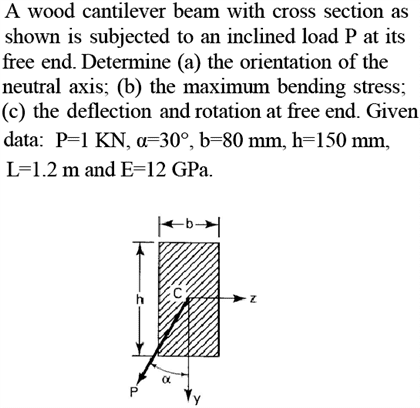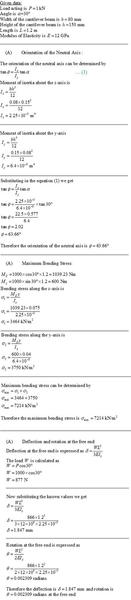# Bending of cantilever beam

• temaire
In summary, the conversation discusses a problem involving the computation of deflection and rotation in a beam. The participant temaire asks for confirmation on whether their work is correct and points out a mistake. They also mention the importance of using the correct unit. The other participant asks about finding the resultant rotation and suggests stating the components instead.

## Homework Statement## The Attempt at a SolutionIs my work correct?

temaire: I do not know if part (a) is correct, because I do not remember the formula. I will let someone else check part (a).

Your answer for part (b) currently looks correct. In part (c), you currently computed only the y-direction deflection and rotation, which are correct. But I think you now might also need to compute the z-direction deflection and rotation.

You accidentally typed 877, instead of 866, although you did not use it.

By the way, kN/m^2 is called kPa. Always use the correct, special name for a unit. E.g., 7214 kPa, not 7214 kN/m^2. However, it is better if you use 7.214 MPa, instead of 7214 kPa.

I've calculated the deflection and rotation of the beam in the z-direction.

I know that the total deflection of the beam is the resultant of the deflections in the y and z directions, as shown

$$\delta = \sqrt{u^2 + v^2}$$

where $u$ is the deflection in the z-direction and $v$ is the deflection in the y-direction.

However, how do I find the resultant rotation? Do I simply use the above formula and just switch $u$ and $v$ with the $\theta_y$ and $\theta_z$?

temaire: Your resultant deflection looks great. Regarding the resultant rotation, we would need to think that over for awhile. I am not sure yet. However, would you settle for just stating the y and z components of rotation? You might not need to compute a resultant rotation. Just state the two components, theta_y and theta_z (?).

Yes, I am leaving my answer for rotation in terms of y and z.

Thanks for the replies.